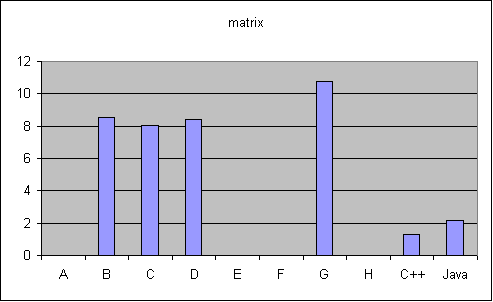# Matrix Computations

Performs a series of matrix computations, N times.  The matrices have square and have size SIZE, which is currently defined as 300.  Vectors are of length SIZE.  There are three matrices, A, B, and C, and two vectors, X and Y.  The implementation of each computation is the naive, unoptimized algorithm.  The computations performed are:

• Initialize each element of all the matrices and vectors.

• 5-pointed update: update A[i] based on the values immediately above, below, left, and right of it in the array.  Used in some Jacobi algorithms.

• 9-pointed update: update A[i] based on all values surrounding it in the array, including the diagonals.  Used in many algorithms, such as image smoothing.

• Matrix-Vector Multiply: Y = A * X

• Matrix-Matrix Multiply: C = A * B

This tests the efficiency of your array bounds checking and null array checking.  If your compiler performs any loop optimizations, performance will greatly improve.  Probably this is why the C++ and Java implementations are so much faster than the Iota ones. Loop unrolling, bounds check elimination, and tiling would be particularly effective.  It also tests the efficiency of integer math.

Source: [matrix.im matrix.cpp Matrix.java]
Input:
N=1
Output:
Y and C == 1202

 C++ 1.32Java 2.15 C 8.05 D 8.41 B 8.54 G 10.75